# Find the interest to the nearest cent Question #1 Amount: \$5432 Annual Interest Rate: 6.2% Time: 3 years Question #2 \$4500 at 5.5% for 4 1/2 years Question #3 \$3680 at 6.75% for 2 1/4 years Question #4 5.5% for 1 3/4 years on \$2543 I'm sorry for so m

Find the interest to the nearest cent

Question #1
Amount: \$5432
Annual Interest Rate: 6.2%
Time: 3 years

Question #2
\$4500 at 5.5% for 4 1/2 years

Question #3
\$3680 at 6.75% for 2 1/4 years

Question #4
5.5% for 1 3/4 years on \$2543

I'm sorry for so many but I just don't understand them =]

You're right for # 2. :-)

It's easiest to use the decimal equivalents for the fractions of a year: 2 1/4 (2.25) and 1 3/4 (1.75).

# 3:

I = 3680 * 0.0675 * 2.25
The basic formula is Interest equals principal times rate times time or I = PRT.

For # 1:
I = 5432 * 0.062 * 3
I = 1010.35

So for #2 the answer is 1113.75. But I still don't understand #3 and #4 because I don't know how to multiply the years. You know 2 1/4 and 1 3/4
I have a question, is the different wording of #4 significant? Because it says 5.5% for 1 3/4 years on \$2543. I am not having any trouble with the others, just this one.
Oh yea! It's like your counting by quarters. Man I forgot. My bad =] Thank You for the great help maybe I might come back for more help today. Again thanks for the great help!!
You're very welcome. And I look forward to helping you again.

##### Add Answer of: Find the interest to the nearest cent Question #1 Amount: \$5432 Annual Interest Rate: 6.2% Time: 3 years Question #2 \$4500 at 5.5% for 4 1/2 years Question #3 \$3680 at 6.75% for 2 1/4 years Question #4 5.5% for 1 3/4 years on \$2543 I'm sorry for so m
Similar Homework Help Questions
• ### Loan interest For the loan amount, interest rate, annual payment, and loan term shown in the...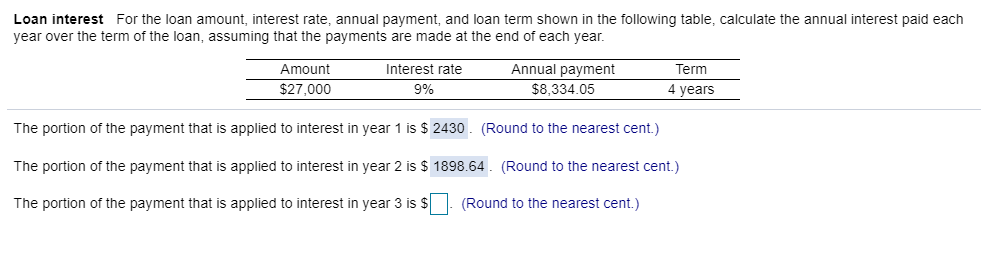Loan interest For the loan amount, interest rate, annual payment, and loan term shown in the following table, calculate the annual interest paid each year over the term of the loan, assuming that the payments are made at the end of each year. Amount Interest rate \$27,0009 % Annual payment \$8,334.05 Term 4 years The portion of the payment that is applied to interest in year 1 is \$2430. (Round to the nearest cent.) The portion of the payment that...

• ### An amount of \$15,000 is borrowed from the bank at an annual interest rate 12% h...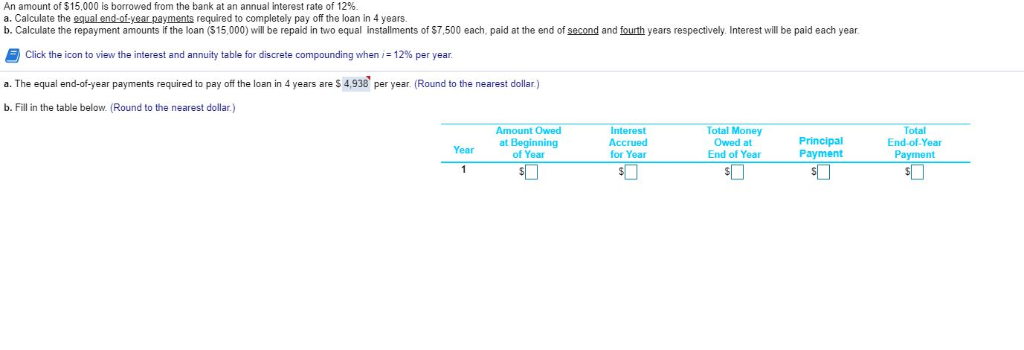An amount of \$15,000 is borrowed from the bank at an annual interest rate 12% h Calculate the repavment amounts if the loan (\$15 000) will be repaid in two equal installments of \$7.500 each, paid at the end of second and fourth years respectively. Interest will be paid each year Click the icon to view the interest and annuity table for discrete compounding when i- 12%% per year . a. The equal end-of-year payments required to pay off the...

• ### QUESTION 1 At an interest rate of 3%, what comes closest to the amount of time...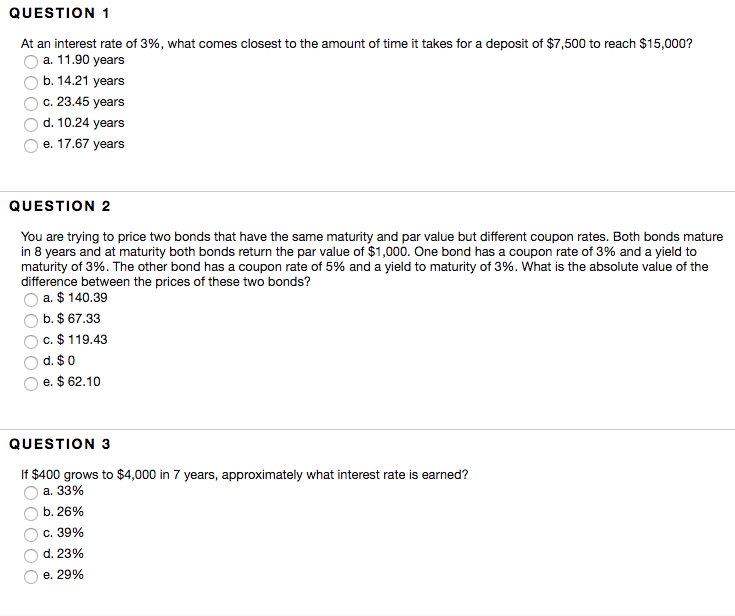QUESTION 1 At an interest rate of 3%, what comes closest to the amount of time it takes for a deposit of \$7,500 to reach \$15,000? O a. 11.90 years b. 14.21 years c. 23.45 years d. 10.24 years e. 17.67 years QUESTION 2 You are trying to price two bonds that have the same maturity and par value but different coupon rates. Both bonds mature in 8 years and at maturity both bonds return the par value of \$1,000....

• ### find the interest for the loan principal rate time \$1,800 6 1/2% 3 3/4 years

find the interest for the loanprincipal rate time\$1,800 6 1/2% 3 3/4 years

• ### quiz: Chapter 4 Quiz (Copy) Time Remaining: 01:01:04 Submit Qu is Question: 1 pt 8 of...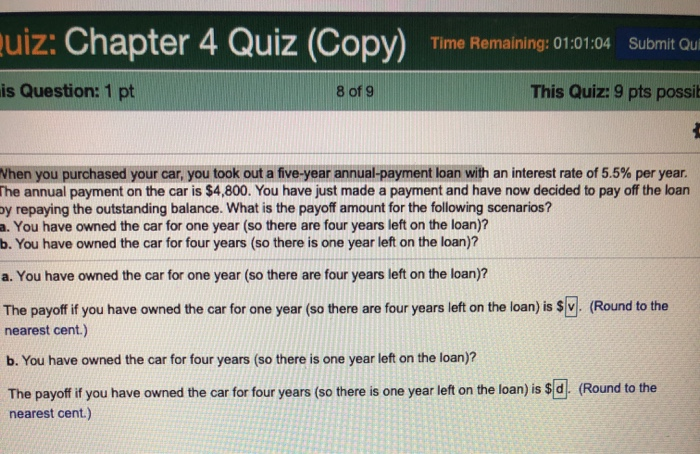quiz: Chapter 4 Quiz (Copy) Time Remaining: 01:01:04 Submit Qu is Question: 1 pt 8 of 9 This Quiz: 9 pts possit When you purchased your car, you took out a five-year annual-payment loan with an interest rate of 5.5% per year. The annual payment on the car is \$4,800. You have just made a payment and have now decided to pay off the loan by repaying the outstanding balance. What is the payoff amount for the following scenarios? a....

• ### sorry for the amount of photos i wanted to make sure the question and its parts...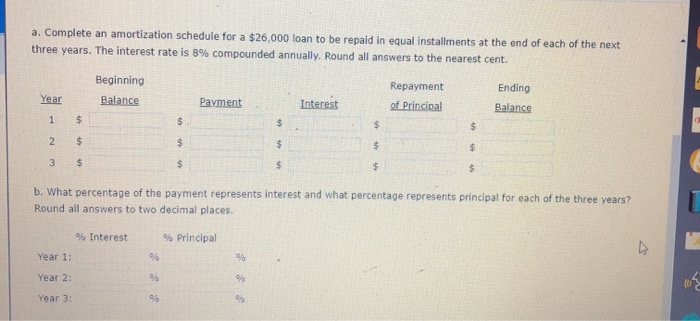sorry for the amount of photos i wanted to make sure the question and its parts were clear. im having trouble with this question, could someone find the answers and explain how they got them? Thanks! a. Complete an amortization schedule for a \$26,000 loan to be repaid in equal installments at the end of each of the next three years. The interest rate is 8% compounded annually. Round all answers to the nearest cent. Beginning Balance Repayment of Principal...

• ### collegelink//course resource/section/7470/assignment/14040 5 Arpandeep Kaur K n 4 (3) n 5 (2) -n 6(1) en 7...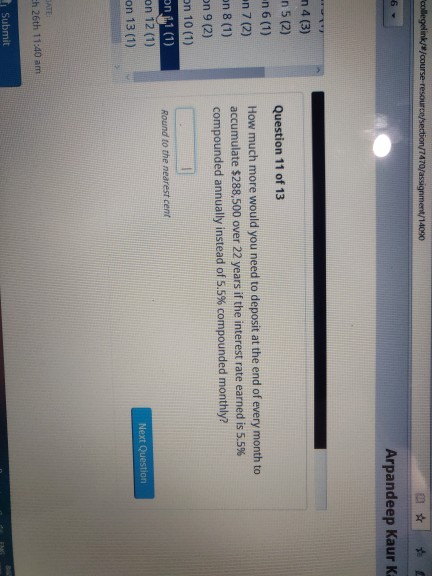collegelink//course resource/section/7470/assignment/14040 5 Arpandeep Kaur K n 4 (3) n 5 (2) -n 6(1) en 7 (2) Question 11 of 13 How much more would you need to deposit at the end of every month to accumulate \$288,500 over 22 years if the interest rate earned is 5.5% compounded annually instead of 5.5% compounded monthly? on 8 (1) on 9 (2) On 10 (1) on 11 (1) on 12 (1) on 13 (1) Round to the nearest cent Next Question...

• ### Quiz: Chapter 4 Quiz (Copy) Time Remaining: 02:33:54 Submit Quiz This Question: 1 pt 1 of 9 This Quiz: 9 pts possib...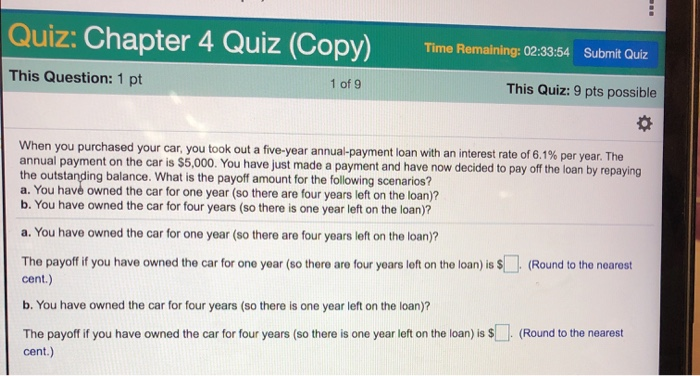Quiz: Chapter 4 Quiz (Copy) Time Remaining: 02:33:54 Submit Quiz This Question: 1 pt 1 of 9 This Quiz: 9 pts possible When you purchased your car, you took out a five-year annual-payment loan with an interest rate of 6.1 % per year. The annual payment on the car is \$5,000. You have just made a payment and have now decided to pay off the loan by repaying the outstanding balance. What is the payoff amount for the following scenarios?...

• ### Please answer question 2, 3 and 4. 2. If your credit card chrries a rate of...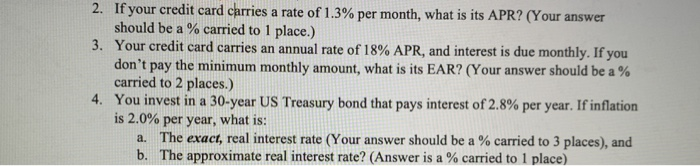Please answer question 2, 3 and 4. 2. If your credit card chrries a rate of 1.3% per month, what is its APR? (Your answer should be a % carried to 1 place.) 3. Your credit card carries an annual rate of 18% APR, and interest is due monthly. If you don't pay the minimum monthly amount, what is its EAR? (Your answer should be a % carried to 2 places.) 4. You invest in a 30-year US Treasury bond...

• ### Loan amortization schedule Personal Finance Problem Joan Messineo borrowed \$46,000 at a 4% annual rate of...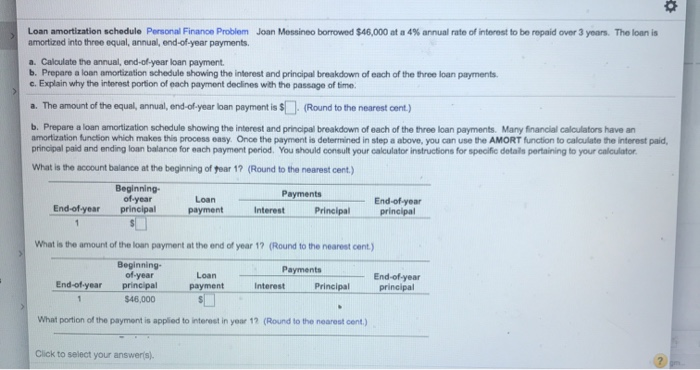Loan amortization schedule Personal Finance Problem Joan Messineo borrowed \$46,000 at a 4% annual rate of interest to be repaid over 3 years. The loan is amortized into three equal, annual end-of-year payments Calculate the annual end of year loan payment b. Prepare a loan amortization schedule showing the interest and principal breakdown of each of the three loan payments. c. Explain why the interest portion of each payment declines with the passage of time. a. The amount of the...

Need Online Homework Help?